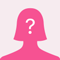# Two angles of a triangle are 32 degrees, and 110 degrees.what is the measurement of the third angle?
+1 y
A. 218

B. 180

C. 142

D. 38

and plz explain why.
Two angles of a triangle are 32 degrees, and 110 degrees.
5
2